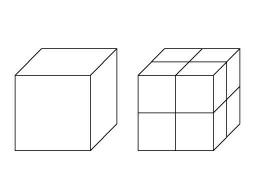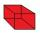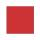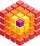# Cube 3

How many times will increase the volume of a cube if we double the length of its edge?

n =  8

### Step-by-step explanation:Did you find an error or inaccuracy? Feel free to write us. Thank you!Tips to related online calculators
Tip: Our volume units converter will help you with the conversion of volume units.

## Related math problems and questions:

• Cube 2How many times will increase if the surface area of the cube if we triple length of its edge?
• CuboidHow many times will increase the volume of a cuboid, if one dimension is twice larger, second dimension three times larger and third dimension four times lower?
• Cube basicsHow long is the edge length of a cube with volume 15 m3?
• CubeHow many times increases the surface area of a cube with edge 23.4 cm if the length of the edge doubled?
• CubeOne cube has an edge increased five times. How many times will larger it's surface area and volume?
• CubeThe cube weighs 11 kg. How weight is a cube of the same material if its dimensions are 3-times smaller?
• Special cubeCalculate the edge of cube, if its surface and its volume is numerically equal number.
• The cubeThe cube has a surface area of 486 m ^ 2. Calculate its volume.
• 3x squareThe side length of the square is 54 cm. How many times increases the content area of a square if the length of side increase three times?
• Cube V2SThe volume of the cube is 27 dm cubic. Calculate the surface of the cube.
• For thinkingsThe glass cube dive into the aquarium, which has a length of 25 cm, width 20 cm and height of 30 cm. Aquarium water rises by 2 cm. a) What is the volume of a cube? b) How many centimeters measure its edge?
• Area of rectangleHow many times will increase the area of the rectangle if we increase twice the length and at the same time we decrease the width by half?
• Mike choseMike chose 4 identical cubes, 3 identical prisms and 2 identical cylinders from the kit. The edge of the cube is 3 cm long. The prism has two dimensions the same as the cube, its third dimension is 2 times longer. The diameter of the base of the cylinder
• Edges or sidesCalculate the cube volume, if the sum of the lengths of all sides is 276 cm.
• The cubeThe cube has a surface of 600 cm2. What is its volume?
• Cube edgesIf the edge length of the cube increases by 50%, how does the volume of this cube increase?
• Tetrahedral prismThe height of a regular tetrahedral prism is three times greater than the length of the base edge. Calculate the length of the base edge, if you know that the prism volume is 2187 cm3.